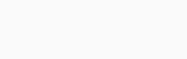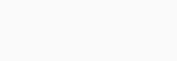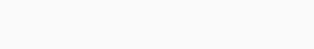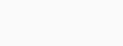## Intro to evaluation metrics for predictive models and how to use them in Spark MLlib

Introduction

Evaluating predictive models is important part of building efficient and accurate predictive models. After a model has been built, one needs to get feedback about performance, make some improvements and repeat those steps until you achieve desirable accuracy. For measuring performance of a model different evaluation metrics are being used depending on model type and implementation plan. At the end of evaluation, our motive should be selecting a model which gives highest accuracy for provided dataset.

When we talk about predictive models, we are talking either about a classification model or a regression model. In this article will be explained what are classification and regression, which evaluation metrics are used for evaluating each type, how those metrics work and what are advantages and disadvantages of using them.

Concrete examples will be provided in Spark MLlib which comes with number of machine learning algorithms and metrics for measuring their performance.

Classification

To begin with, classification is a supervised learning problem that identifies to which category a new observation belongs on the basis of a training dataset. There are different types of classification algorithms like binary classification, multiclass classification, multilabel classification and ranking systems. The simplest of them is binary classification where there are only 2 possible categories for each data point (e.g. deciding if phone call is “fraud” or “not fraud”). All evaluation metrics for classification will be explained on that model for easier understanding.

For each classification model and for each data point there is a true output and a predicted output. Because of that, the results can be assigned to one of four categories:

1)    True Positive (TP) – label is positive and prediction is also positive

2)    True Negative (TN) – label is negative and prediction is also negative

3)    False Positive (FP) –  label is negative but prediction is positive

4)    False Negative (FN) – label is positive but prediction is negativeDepending on those categories we can calculate different evaluation metrics like precision and recall, F-measure, Receiver Operating Characteristic (ROC) and Area Under ROC Curve which will be explained in next section.

Classification evaluation metrics

a)    precision and recall

Precision (Positive Predictive Value) answers how many selected items from dataset are relevant while recall (sensitivity or True Positive Rate) answers how many relevant items are selected. Formulas for calculating both measures are simple and intuitive.Let’s suppose we have a dataset containing 100 records holding information about phone calls containing 12 fraud phone calls. Also, let’s suppose an algorithm for recognizing frauds identifies 8 fraud phone calls, 6 are actually frauds (true positives) while the rest are non-frauds (false positives). By using mentioned formulas we can calculate precision (PPV = 6/8 = 0.75) and recall (TPR = 6/12 = 0.5). From given example we see that high precision means that an algorithm returned more relevant results than irrelevant ones (from 8 identified frauds he returned 6 that are really frauds), while low recall means that an algorithm didn’t return most of the relevant results (there are 12 frauds in dataset but algorithm identified only 6 of them).

It is important to say that there is often an inverse relationship between precision and recall, where it is possible to increase one at the cost of reducing the other.

b)    F measure

F-measure is a measure of a model’s accuracy. It considers both the precision P and the recall R to compute the score. The F1 score is the harmonic mean of the precision and recall, where an F1 score reaches its best value at 1 (perfect precision and recall) and worst at 0.Sometimes, better results are obtained when recall and precision are not evenly weighted. For that case we use this formula:ROC is a performance measure that is based on the True Positive Rate (TPR) and the False Positive Rate (FPR). FPR can be calculated as (FP / (FP + TN)).

The ROC space uses FPR on the x-axis and TPR on the y-axis. Each prediction result represents one point in the ROC space. The perfect classification is a point in the upper left corner or coordinate (0,1), where there are no false positives or false negatives. A random guess gives a point along a diagonal line which divides the ROC space. Points above the diagonal represent good classification results and points below the line represent bad results. A ROC curve is created by connecting all ROC points of a classifier in the ROC space.Another advantage of using the ROC plot is a single measure called the AUC (area under the ROC curve). It is an area under the curve calculated in the ROC space. Range of AUC score is between 0 and 1 but the actual scores of good classifiers are greater than 0.5.Classification evaluation metrics (Spark MLlib example)

The following code snippet in Java shows how to train a binary classification algorithm on the predefined data and evaluate the performance of the algorithm with binary evaluation metrics mentioned earlier.

// Split initial dataset into training (60%) and testing (40%)
JavaRDD<LabeledPoint>[] splits = data.randomSplit(new double[]{0.6,0.4},11L);
JavaRDD<LabeledPoint> training = splits.cache();
JavaRDD<LabeledPoint> test = splits;
// Run training algorithm to build the model.
LogisticRegressionModel model = new LogisticRegressionWithLBFGS()
  .setNumClasses(2)
  .run(training.rdd());
// Clear the prediction threshold so the model will return probabilities
model.clearThreshold();
// Compute raw scores on the test set.
JavaPairRDD<Object, Object> predictionAndLabels = test.mapToPair(p ->
  new Tuple2<>(model.predict(p.features()), p.label()));
// Get evaluation metrics.
BinaryClassificationMetrics metrics =
  new BinaryClassificationMetrics(predictionAndLabels.rdd());
// Precision by threshold
JavaRDD<Tuple2<Object, Object>> precision = metrics.precisionByThreshold().toJavaRDD();
System.out.println("Precision by threshold: " + precision.collect());
// Recall by threshold
JavaRDD<?> recall = metrics.recallByThreshold().toJavaRDD();
System.out.println("Recall by threshold: " + recall.collect());
// F1 Score by threshold
JavaRDD<?> f1Score = metrics.fMeasureByThreshold().toJavaRDD();
System.out.println("F1 Score by threshold: " + f1Score.collect());
// F2 Score by threshold
JavaRDD<?> f2Score = metrics.fMeasureByThreshold(2.0).toJavaRDD();
System.out.println("F2 Score by threshold: " + f2Score.collect());
// ROC Curve
JavaRDD<?> roc = metrics.roc().toJavaRDD();
System.out.println("ROC curve: " + roc.collect());
// AUROC
System.out.println("Area under ROC = " + metrics.areaUnderROC());

Regression

Regression analysis is used when predicting a continuous output variable (dependent variable) from a number of predictors (independent variables). It helps us understand how the output changes when any of the independent variables is varied and does a set of predictor variables do a good job in predicting an outcome.

Regression function is a function of the independent variables and the simplest form of that function is defined by the formula y = c + b*x, where y is estimated output, c is constant, b is regression coefficient, and x is score on the independent variable.There are multiple evaluation metrics for regression analysis that will be explained in the following section.

Regression evaluation metrics

a)    Mean Squared Error (MSE)

Mean squared error tells us how close a regression line is to our dataset. It does it by calculating distances (errors) from the points of dataset to regression line and squaring them. After that, it calculates average of those distances. It is a measure of quality of a predictor – it is always non-negative, and values closer to zero are better.

If {\displaystyle {\hat {Y}}}Y’ is a vector of {\displaystyle n}n predictions, and {\displaystyle Y}Y is the vector of observed values of the variable being predicted, then the MSE of the predictor is computed as:It is important to say that mean squared error has the disadvantage of heavily weighting outliers (points which are far away from expected values).

b)    Root Mean Squared Error (RMSE)

Root mean squared error is the standard deviation of prediction errors. It is a measure of how spread out prediction errors are. In other words, it tells you how concentrated the data is around the line of best fit. Like MSE, it is sensitive to outliers meaning larger errors have a disproportionately large effect on RMSE. Formula for calculating RMSE is shown below:c)    Mean Absolute Error (MAE)

Mean absolute error is a measure of difference between predicted and observed variables. It is an average of the absolute errors and{\displaystyle |e_{i}|=|y_{i}-x_{i}|} it is simpler and more interpretable than MSE or RMSE because it does not require the use of squares or square roots. Also, each error contributes to MAE in proportion to the absolute value of the error, which is not true for MSE or RMSE where outliers have large effect on outcome. Formula for calculating MAE is shown below:d)    Coefficient of Determination (R2)

Coefficient of determination is a measure of how close the data is to the fitted regression line. If the distance between regression line and data is small, R2 has value close to 1, meaning model is reliable for future predictions. On the other hand, if R2 value is close to 0 it indicates that model fails to accurately fit the data. R2 does not indicate whether the independent variables are a cause of the changes in the output or if the most appropriate set of independent variables has been chosen.

Formula for calculating R2 is shown below:e)    Explained Variance

Explained variance measures the proportion to which a mathematical model accounts for the variation (dispersion) of a given data set. Formula for calculating explained variance is shown below:Regression evaluation metrics (Spark MLlib example)

Following code snippet in Java shows how to train a linear regression algorithm on the predefined data and evaluate the performance of the algorithm with regression evaluation metrics mentioned earlier.

// Building the model

int numIterations = 100;

LinearRegressionModel model = LinearRegressionWithSGD.train(JavaRDD.toRDD(parsedData), numIterations);

// Evaluate model on training examples and compute training error

JavaPairRDD<Object, Object> valuesAndPreds = parsedData.mapToPair(point ->

new Tuple2<>(model.predict(point.features()), point.label()));

// Instantiate metrics object

RegressionMetrics metrics = new RegressionMetrics(valuesAndPreds.rdd());

// Squared error

System.out.format(“MSE = %f\n”, metrics.meanSquaredError());

System.out.format(“RMSE = %f\n”, metrics.rootMeanSquaredError());

// R-squared

System.out.format(“R Squared = %f\n”, metrics.r2());

// Mean absolute error

System.out.format(“MAE = %f\n”, metrics.meanAbsoluteError());

// Explained variance

System.out.format(“Explained Variance = %f\n”, metrics.explainedVariance());

Written by:

Ivan ćirić

ivan.ciric@multicom.hr